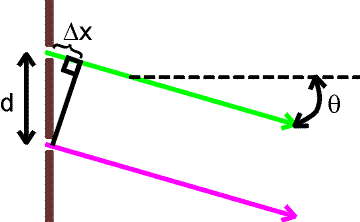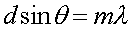Two-slit interference The simplest and most illustrative example of two-slit interference is two-slit interference. For this case we consider monochromatic (of one wavelength) light normally incident on two slits as shown below.The violet and green wave crests originate from the lower and upper slits respectively. In the directions shown by the blue lines, the violet and green waves rise and fall together. This is called constructive interference. Midway between the angles that give maxima, the two contributions are 180 degrees out of phase, and tend to nearly cancel one another. The result is that if a screen is placed to the right, an interference pattern results with peaks in the brightness in the direction of the blue arrows.One can solve for the angular position of the maxima. Consider two rays emited at an angle q from each of the slits. These nearly parallel rays come together at a screen far away. If the distance Dx is and integral number m of wavelengths, a bright maximum will occur on the screen.The above expression gives the angles for the m = 0,1,2... maxima illustrated above.